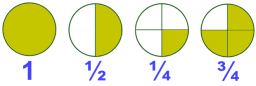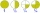# Product and sum

What is the product of two fourths  and the sum of three halves and four?

x =  2.75

### Step-by-step explanation:Did you find an error or inaccuracy? Feel free to write us. Thank you!Tips to related online calculators
Need help to calculate sum, simplify or multiply fractions? Try our fraction calculator.

## Related math problems and questions:

• Translate 2Translate the given phrases to mathematical phrases. Thrice the sum of three fifths and two thirds less one half is what number?
• Product of the sum and differenceCalculate the product of the sum and difference of numbers -7 and -2.
• Product increasedWhen the product of 2/3 and 6/10 is increased by 2/5, the result is?
• Complicated sum minus productWhat must be subtracted from the sum of 3/8 and 5/16 to get difference equal to the product of 5/8 and 3/16?
• Sum of 20Sum of the two numbers is 15 1/6. If one of the numbers is 2 2/3, find the other number.
• Quotient and productIf the quotient of [8/5 divided by 8/10] is added to the product of [8/14 x 7/12 x 3/8], what is the sum?What is the sum of 2/3 and 3/10?
• Two numbers and its productThe product of two numbers are 2/3. If on of them is 1/10, what is the other?To three-eighths of one third, we add five quarters of one half and multiply the sum by four. How much will we get?
• Sum three fractionsWork out the sum of 1/4, 1/5 and 3/10.Add two mixed fractions: 2 4/6 + 1 3/6The sum of 3 mixed numbers is 20 13/15. two of the numbers are 6 1/3 and 7 5/6. what is the third number?If the quotient of 8/13 and 2 is subtracted from the product of 1 3/4 and 8/21, what is the difference?Multiply the quotient of 6 and 2 by 3, then add 1. Add 1 and 6, then divide by 2 and multiply by 3. Divide 6 by the product of 2 and 3, then add 1.Write a real-world problem involving the multiplication of a fraction and a whole number with a product that is between 8 and 10, then solve the problem.Product of two fractions is 9 3/5 . If one of the fraction is 9 3/7. Find the other fraction.7 is added to the sum of 4/5 and 6/7